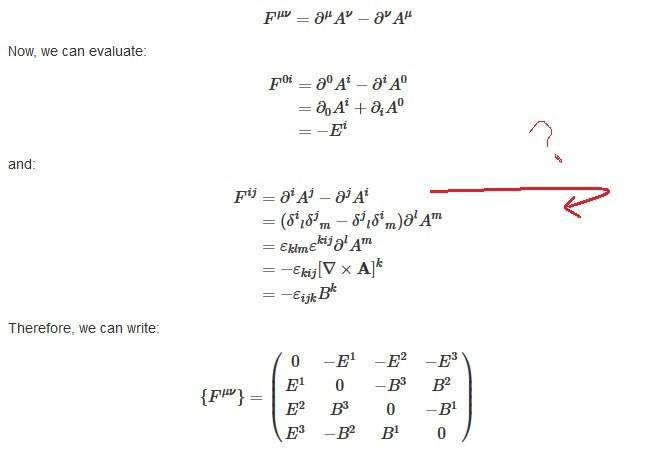# Deriving the electromagnetic field strength tensor

• rwooduk

#### rwooduk

Just one last question today if someone can help. I'm trying to derive the electromagnetic field strength tensor and having a little trouble with (i think) the use of identities, please see below:I understand the first part to get -Ei, but it's the second line of the next bit I don't understand. I see he wants to get line one to the form he has done so he can use the following identity:

##\varepsilon _{ijk}\varepsilon _{ilm} = \delta _{jl}\delta _{km} - \delta _{jm}\delta _{kl}##

But I'm unsure how he has got there and line 2 to 3 looks a little iffy. I've tried using ##\delta _{ij}\delta _{jk} = \delta _{ik}## but that didn't go very well, and also I've always thought something of the form ##\delta_{i}^{l} ## was the kroneka delta function, so I'm unsure how it would come in here.

If someone can help by putting a couple of inbetween stages in the derivation it would really help my understanding of what is going on and the notation / identities he's used.

## \delta^{i}{}_l\delta^{j}{}_m \partial^lA^m= \partial^iA^j##

etc.

•rwooduk
Thanks for the reply, I can see that relation by looking at the difference between the lines, but how did he get the ## \partial^iA^j## term to equal that?
thanks again

The sum over ##m## when there is a ##\delta_i^m## is non-zero only when ##i = m## and you can replace the ##m## sum by simply replacing the other ##m## by the ##i## and removing the delta.

•rwooduk
The sum over ##m## when there is a ##\delta_i^m## is non-zero only when ##i = m## and you can replace the ##m## sum by simply replacing the other ##m## by the ##i## and removing the delta.

ahh ok i see so j must = m and i must = m so it goes to Am. And likewise i must = l and j must = l therefore it goes to delta l. This is probably a stupid question, but wouldn't you then get zero in the bracket? i.e. (1-1)

This is probably a stupid question, but wouldn't you then get zero in the bracket? i.e. (1-1)
No. In one of the terms the i replaces the l and in the other it replaces the m. This gives different terms.

•rwooduk
No. In one of the terms the i replaces the l and in the other it replaces the m. This gives different terms.

hmm, kind of got that, ok thanks, will work on this some more.

Thanks for all the replies.

Isn't this way too complicated? I usually write
$$F^{ij}=\partial^{i} A^{j} - \partial^{j} A^i=-(\partial_i A^j-\partial_{j} A^{i})=-\epsilon^{ijk} B^{k},$$
where one has to keep in mind that
$$\partial^i=-\partial_i=\frac{\partial}{\partial x^i}$$
and then use that
$$\vec{B}=\vec{\nabla} \times \vec{A}.$$

•rwooduk
-(\partial_i A^j-\partial_{j} A^{i})=-\epsilon^{ijk} B^{k},

do you go from LHS to RHS from memory, or are you saying you used the things you say below it? It's the steps inbetween I'm interested in.

would welcome a simpler 'derivation' of this step though

This is simply the definition of the curl operation and the vector potential by ##\vec{B}=\vec{\nabla} \times \vec{A}##.

•rwooduk